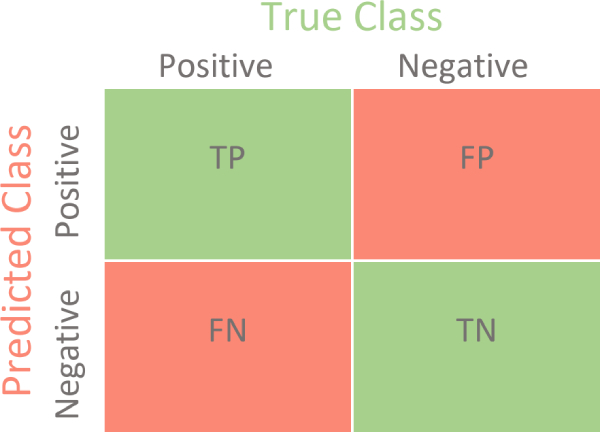• +91-9872993883
• +91-8283824812
• info@ris-ai.com

# Confusion Matrix in Python ¶

In this article , you'll see a full example of a Confusion Matrix in Python. A Confusion Matrix is an N x N matrix used for evaluating the performance of a classification model, where N is the number of target classes. The matrix compares the actual target values with those predicted by the Machine Learning model. This gives us a holistic view of how well our classification model is performing and what kinds of errors it is making.1. The target variable has two values: Positive or Negative
2. The columns represent the actual values of the target variable
3. The rows represent the predicted values of the target variable

## Understanding True Positive, True Negative, False Positive and False Negative in a Confusion Matrix ¶

1. True Positive (TP)

The predicted value matches the actual value. The actual value was positive and the model predicted a positive value

2. True Negative (TN)

The predicted value matches the actual value. The actual value was negative and the model predicted a negative value

3. False Positive (FP) – Type 1 error

The predicted value was falsely predicted. The actual value was negative but the model predicted a positive value Also known as the Type 1 error

4. False Negative (FN) – Type 2 error

The predicted value was falsely predicted. The actual value was positive but the model predicted a negative value Also known as the Type 2 error

## Precision vs. Recall¶

#### Precision tells us how many of the correctly predicted cases actually turned out to be positive. ¶

Precision Formuls:

              TruePositives / (TruePositives + FalsePositives)



This would determine whether our model is reliable or not.

#### Recall tells us how many of the actual positive cases we were able to predict correctly with our model. ¶

Recall Formula:

             TruePositives / (TruePositives + FalseNegatives)### F1-score¶

F1-score is a harmonic mean of Precision and Recall, and so it gives a combined idea about these two metrics. It is maximum when Precision is equal to Recall.

But there is a catch here. The interpretability of the F1-score is poor. This means that we don’t know what our classifier is maximizing – precision or recall? So, we use it in combination with other evaluation metrics which gives us a complete picture of the result.

F1 formula:

            F-Measure = (2 * Precision * Recall) / (Precision + Recall)



#### Confusion Matrix using scikit-learn in Python ¶

In :
# confusion matrix in sklearn
from sklearn.metrics import confusion_matrix
from sklearn.metrics import classification_report

# actual values
actual = [1,0,0,1,0,0,1,0,0,1]
# predicted values
predicted = [1,0,0,1,0,0,0,1,0,0]

# confusion matrix
matrix = confusion_matrix(actual,predicted, labels=[1,0])
print('Confusion matrix : \n',matrix)

# outcome values order in sklearn
tp, fn, fp, tn = confusion_matrix(actual,predicted,labels=[1,0]).reshape(-1)
print('Outcome values : \n', tp, fn, fp, tn)

# classification report for precision, recall f1-score and accuracy
matrix = classification_report(actual,predicted,labels=[1,0])
print('Classification report : \n',matrix)

Confusion matrix :
[[2 2]
[1 5]]
Outcome values :
2 2 1 5
Classification report :
precision    recall  f1-score   support

1       0.67      0.50      0.57         4
0       0.71      0.83      0.77         6

accuracy                           0.70        10
macro avg       0.69      0.67      0.67        10
weighted avg       0.70      0.70      0.69        10


In [ ]: